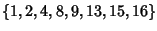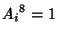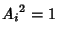## Finite Group Z8One of the three Abelian groups of the five groups total of Order 8. An example is the residue classes modulo 17 which Quadratic Residues, i.e.,under multiplication modulo 17. The elementssatisfy, four of them satisfy, and two satisfy. The Cycle Graph is shown above.

See also Finite Group D4, Finite Group Q8, Finite Group Z2Z4, Finite Group Z2Z2Z2